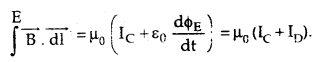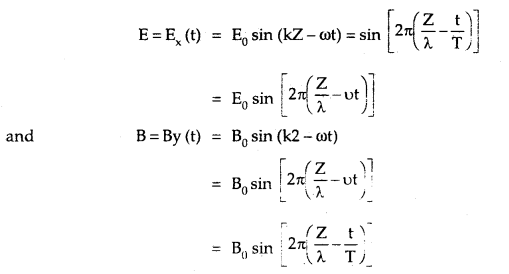By going through these CBSE Class 12 Physics Notes Chapter 8 Electromagnetic Waves, students can recall all the concepts quickly.

## Electromagnetic Waves Notes Class 12 Physics Chapter 8

→ Displacement current is a 1 ways equal to charging (for discharging) current and lasts so long as the capacitor (producing varying electric field) is charged or discharged.

→ An accelerated charged particle emits e.m. waves.

→ $$\overrightarrow{\mathrm{S}}$$ = $$\overrightarrow{\mathrm{E}}$$ × $$\overrightarrow{\mathrm{B}}$$ is called Poynting vector acts in a direction perpendicular to the plane of $$\overrightarrow{\mathrm{E}}$$ and $$\overrightarrow{\mathrm{B}}$$ .

→ The displacement current is named so because it is produced by the displacement of electrons caused by changing electric fields.

→ X-rays have the shortest wavelength (≈ 1 Å).

→ The charging or discharging current is called conduction current.

→ The amplitude of electric and magnetic fields in free space in e.m. waves are related as E = CB

→ Electric vector is called light vector as it is responsible for the optical effect of e.m. wave.

→ The energy of the e.m. wave is shared equally between the electric field vector and the magnetic field vector.

→ Microwaves are very commonly used in radar to locate flying objects like airplanes, jet planes, etc.

→ Tire earth’s atmosphere produces Green House effect. In the absence of the earth’s atmosphere, the temperature on earth during the day will increase and during the night it would decrease.

→ The ozone layer which is present in the stratosphere protects the earth from high-energy radiations coming from outer space.

→ The velocity of em. waves in a medium is given by
v = $$\frac{1}{\sqrt{\mu_{0} \varepsilon_{0} \mu_{\mathrm{r}} \varepsilon_{\mathrm{r}}}}=\frac{C}{\sqrt{\mu_{\mathrm{r}} \varepsilon_{\mathrm{r}}}}$$

→ There is no conduction current in a traveling e.m. wave.

→ Earth’s atmosphere is transparent to visible light and most of the infrared rays are absorbed by the atmosphere.

→ Radio waves were discovered by Hertz and are used in communication.

→ e.m. waves are transverse in nature.

→ e.m. waves exert pressure on the objects on which they fall as they carry energy and momentum.

→ The wavelength range of em. waves are from 10-15 m to 109 m and the frequency range is 1024 Hz to 1 Hz.

→ Green House Effect takes place due to the heating of the earth’s atmosphere due to the trapping of infrared rays by the CO2 layer in the atmosphere.

→ Modified Ampere Circuital law: It states that the line integral of the magnetic field around a closed path is always equal to μ0 times the sum of the conduction dnd displacement currents i.e.,→ Displacement Current: It is defined as the current produced in a region where a change of electric flux takes place due to the change in electric field intensity in that region.

Important Formulae

→ Amper’s circuital law states that
∫ $$\overrightarrow{\mathrm{B}}$$.$$\overrightarrow{\mathrm{dl}}$$ = μ0 IC
where IC = conduction current Displacement current is given by

→ Displacement current is given by
ID = ε0 $$\frac{\mathrm{d} \phi_{\mathrm{E}}}{\mathrm{dt}}$$

→ C = $$\frac{E_{0}}{B_{0}}=\frac{1}{\sqrt{\mu_{0} \varepsilon_{0}}}$$

→ Energy density of electric field, UE = $$\frac{1}{2}$$ ε0 E2

→ Energy density of electric field, UB = $$\frac{\mathrm{B}^{2}}{2 \mu_{0}^{2}}$$

→ Intensity of e.m. wave is given by
I = average energy density × speed of e.m. wave
= $$\frac{1}{2}$$ ε0E2 × C = ρ/4πr²

→ $$\overrightarrow{\mathrm{B}}$$ at a point between the plates of the capacitor at a distance r from its axis is given by.
B = $$\frac{\mu_{0} \mathrm{Ir}}{2 \pi \mathrm{R}^{2}}$$
Where R = radius of each circular plate of the capacitor.

→ Velocity of e.m. waves is
C = vλ

→ An electromagnetic wave of frequency v, wavelength λ propagating along the z-axis, we have→ The speed of light or of electromagnetic waves in a material medium is given by
υ = $$\frac{1}{\sqrt{\mu \varepsilon}}$$
where μ is the permeability of the medium and ε is its permittivity.

→ Bmax = $$\frac{\mu_{0} I_{D}}{2 \pi R}$$